### States of Matter - Gases and Liquids - Revision Notes

CBSE Class 12 Chemistry

Quick Revision Notes
Chapter 1
The Solid State

Solid: Solid is a state of matter in which the constituting particles are arranged very closely.The constituent particles can be atoms, molecules or ions.

Properties of solids:

1. They have definite mass, volume and shape.
2. They are compressible and rigid.
3. Intermolecular distances are very short and hence the intermolecular forces are strong.
4. Their constituent particles have fixed position. sand can only oscillate about their mean positions.

Classification of on the basis of the arrangement of constituent particles: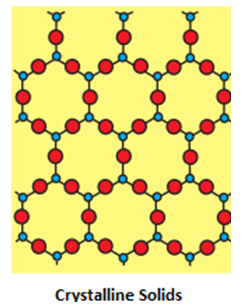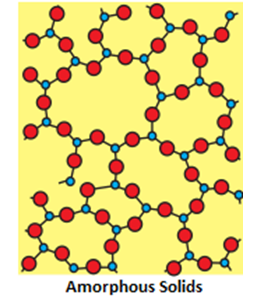• Properties of crystalline solids:
• They have a definite geometrical shape.
• They have a long range order.
• They have a sharp melting point.
• They are anisotropic in nature i.e. their physical properties show different values when measured along different directions in the same crystal.
• They have a definite and characteristic heat of fusion.
• They are called true solids.
• When cut with a sharp edged tool , they split into two pieces and the newly generated surfaces are plain and smooth.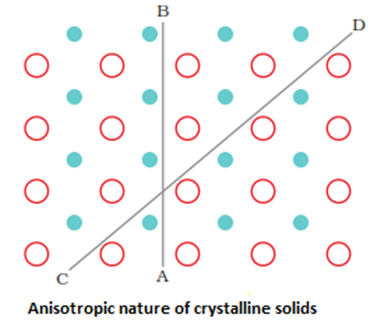• Polymorphic forms or polymorphs:

The different crystalline forms of a substance are known as polymorphic forms or polymorphs .For example: graphite and diamond.

• Characteristics of amorphous solids:
1. They have an irregular shape.
2. They have a short range order.
3. They gradually soften over arrange of temperature.
4. They are isotropic in nature i.e. their physical properties are the same in all directions.
5. When cut with a sharp edged tool, they cut into two pieces with irregular surfaces.
6. They do not have definite heat of fusion.
7. They are called pseudo solids or super cooled liquids. This is because they have a tendency to flow,though very slowly.
• Types of crystalline solids:

A. Molecular Solids

Constituent Particles: Molecules

 Type of solid Constituent Particles Bonding/Attractive Forces Electrical conductivity physical nature Melting point Examples Non-polar solids Molecules Dispersion or London forces Insulator Soft Very low Ar, CCl4, H2, l2, CO2 Polar solids Molecules Dipole- dipole interactions Insulator Soft low HCl, solidSO2, solid NH3 Hydrogen bonded Molecules Hydrogen bonding Insulator Hard low H2O (ice)

B. Ionic Solids

Constituent Particles: Ions
Bonding/Attractive Forces: Coulombic or Electrostatic
Electrical Conductivity: Insulators in solid state but conducts in molten state and in aqueous solutions
Physical Nature: Hard but brittle
Melting Point: High
Examples: $Ca{F}_{2}$, ZnS, MgO, NaCl

C. Metallic Solids
Constituent Particles:
Positive ions in a sea of delocalized electrons
Bonding/Attractive Forces: Metallic bonding
Electrical Conductivity: Conductors in solid state as well as in molten state
Physical Nature: Hard but malleable and ductile
Melting Point: Fairly high
Examples: Fe ,Cu, Ag, Mg

D. Covalent or NetworkSolids
Constituent Particles:
Atoms
Bonding/Attractive Forces: Covalent bonding
Electrical Conductivity: Conductors in solid state as well as in molten state
Physical Nature: Hard but malleable and ductile
Melting Point: Fairly high
Examples: $SiO2$, (quartz), SiC, C (diamond), C(graphite)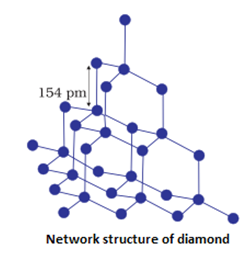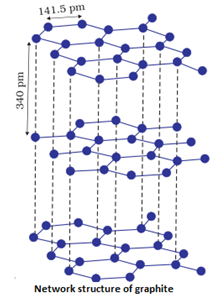• Crystal lattice: A regular ordered arrangement of constituent particles in three dimensions is called crystal lattice.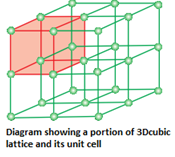• Lattice points or lattice sites:the fixed positions on which the constituent particles are presentare called lattice points or lattice sites. A group of lattice points which when repeated over and over againin3dimensions give the complete crystal lattice.
• Unit cell: It is defined as the smallest repeating unit in space lattice which when repeated over and over again generates the complete crystal lattice. The crystal can consist of an infinite number of unit cells.
• Parameters which characterize a unit cell:
1. Dimensions of the unit cell along the three edges ,a, b and c:these edges may or may not be mutually perpendicular.
2. Inclination of the edges to each other:this is denoted by the angle between the edges $\alpha$,$\beta$ , andrespectively.$\alpha$isthe angle between the edges b and c,$\beta$isthe angle between the edges a and c ,and$\gamma$is the angle between a and b.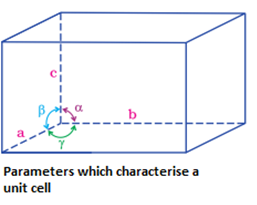• Seven crystal systems:
1. Cubic: $\alpha$=$\beta$=$\gamma$=90° ,a=b=c
2. Tetragonal: $\alpha$=$\beta$=$\gamma$=90° ; a=b$\ne$c
3. Orthorhombic: $\alpha$=$\beta$=$\gamma$=90°; a$\ne$b$\ne$c
4. Monoclinic: $\alpha$=$\gamma$=90°,$\beta$$\ne$90°; a$\ne$b$\ne$c
5. Hexagonal: $\alpha$=$\beta$=90°,$\gamma$=120°; a=b$\ne$c
6. Rhombohedral or trigonal: $\alpha$$\beta$=$\gamma$$\ne$90°;a=b=c
7. Triclinic: $\alpha$$\ne$$\beta$$\ne$$\gamma$$\ne$90°;a$\ne$b$\ne$c
• Types of unit cells:
1. Primitive or simple unit cells have constituent particles only at its corners.
2. Centered unit cells are those unit cells in which one or more constituent particles are present at positions in addition to those present at the corners.
• Types of centered unit cells:
1. Face centered unit cell: It consists of one constituent particle present at the centre of each face in addition to those present at the corners.
2. Body centered unit cell: It consists of a one constituent particle is present at its body centre in addition to those present at the corners.
3. End centered unit cell: It consists of one constituent particle present at the centre of any two opposite faces in addition to those present at the corners.
• Number of particles at different lattice positions: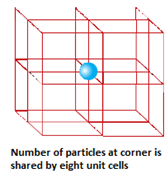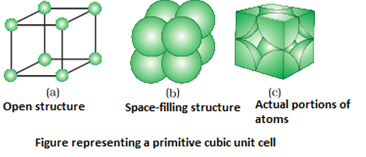• Face centre: if an atom is present at the centre of the face, it is shared by two unit cells. So, only half of the atom actually belongs to the unit cell.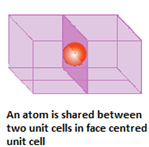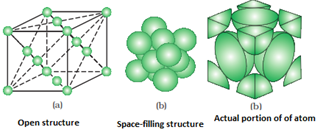• Body centre: if an atom is present at the body centre, it is not shared by any other unit cell.So,that one atom completely belongs to the same unit cell.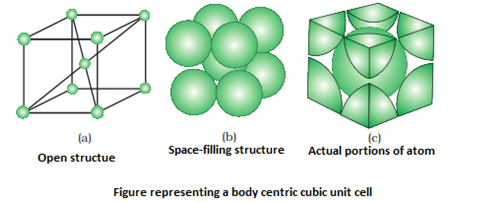• End centre: f an atom is present at the edge centre, it is shared by four unit cells. So, only one fourth of an atom belongs to the unit cell.
• Number of atoms in different unit cells:
1. Primitive unit cell have 1atom
2. Face centered unit cell have 3 atoms
3. Body centered unit cell have 2atoms
• Coordination number: Coordination number is the number of nearest neighbours of a particle.
• Close packed structures: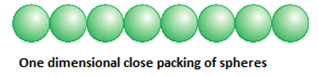• Close packing in two dimensions: It is generated by stacking the rows of close packed spheres in two ways:

i) Square close packing and   ii) Hexagonal close packing.

• Close packing in three dimensions: They can be obtained by stacking the two dimensional layers one above the other. It can be obtained in two ways:

i) Square close packed layers and   ii) Hexagonal close packed layers.

• Square close packing: Here, the spheres of the second row ware placed exactly above those of the first row. This way the spheres are aligned horizontally as well as vertically. The arrangement is AAA type. The coordination number is 4.• Hexagonal close packing: Here, these spheres of these bond row are placed above the first one in as taggered manner in such a way that its spheres fit in the depression of the first row. The arrangement is ABAB type. The coordination number is 6.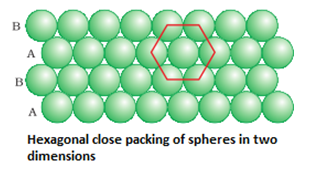• Three dimensional close packing from two dimensional square close packed layers: Here , the spheres of the upper layer are placed exactly over the first layer such the spheres of the layers are perfectly aligned horizontally and vertically.It has a AAAA type pattern. The lattice is simple cubic lattice.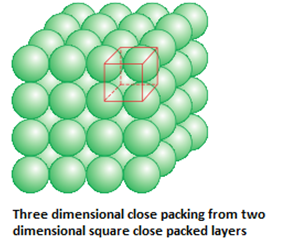• Three dimensional close packing from two dimensional hexagonal close packed layers: There are two steps involved as:
1. Placing these bond layer over the first layer
2. Placing the third layer over the second layer

There are two possibilities: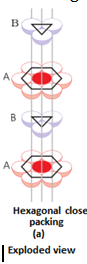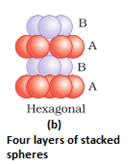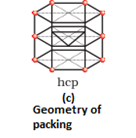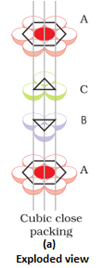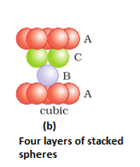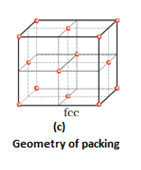• Covering the octahedral voids: Here, octahedral voids of these bond layer may be covered by the spheres of the third layer. It gives rise to ABCABCABC type pattern. The three dimensional structure is called cubic close packed structure or face centered cubic structure. The coordination number is 12.Example: Cu, Ag.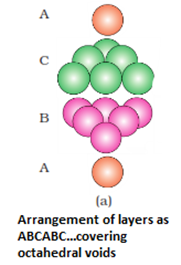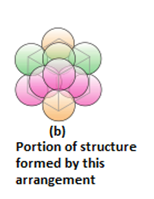• Types of voids: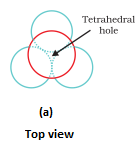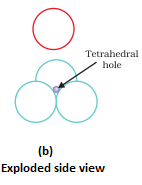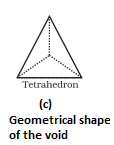• Octahedral void- It is formed at the centre when six spheres are joined in the form of an octahedron.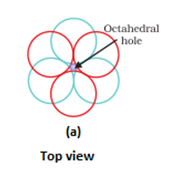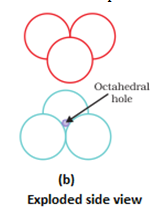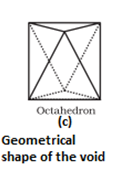• In hexagonal close packing or cubic close packing arrangement, the octa hedral and tetrahedral voids are present. The number of octahedral voids present in a lattice is equal to the number of close packed particles. The number of tetrahedral voids is twice the number of octahedral voids.

For example:
If the number of close packed particles = n
Number of particles present in octahedral voids = n
Then, the number of particles present in tetrahedral voids = 2n

• Packing efficiency: It is the percentage of total space occupied by constituent particles (atoms, molecules orions).

$\text{Packing Efficiency}=\frac{\text{Volume occupied by spheres}}{\text{Total volume of unit cell}}×100$

• Packing efficiency for face centered unit cell =74%
• Packing efficiency for body centered cubic unit cell =68%
• Packing efficiency for simple cubic unit cell =52.4%
• Radius ratio in an octahedral void: For an atom to occupy an octahedral void, its radius must be 0.414 times the radius of the sphere.

• Radius ratio for tetrahedral void: For an atom to occupy a tetrahedral void, its radius must be 0.225 times the radius of the sphere.

• Density of a unit cell is same as the density of the substance.
• Relationship between radius of constituent particle(r) and edge length(a):
1. Simple cubic unit cell: a=2r
2. Face centered unit cell: a=$2\sqrt{2r}$
3. Body centered unit cell: a= $\frac{4r}{\sqrt{3}}$
• Volume of a unit cell=(edge length)3=$a3$
1. Simple cubic unit cell: Volume=$\left(2r\right)3$
2. Face centered unit cell: Volume=${\left(2\sqrt{2r}\right)}^{3}$
3. Body centered unit cell: Volume=${\left(\frac{4r}{\sqrt{3}}\right)}^{3}$
• Number of atoms in a unit cell(z):
1. Simple cubic unit cell: z = 1
2. Face centered unit cell: z = 4
3. Body centered unit cell: z = 2
• Density of unit cell =
• Crystal defects are basically irregularities in the arrangement of constituent particles.
• Types of defects:
1. Point defects- Point defects are the irregularities or deviations from ideal arrangement around a point or an atom in a crystalline substance.
2. Line defects- Line defects are the irregularities or deviations from ideal arrange ment in entire rows of lattice points.
• Different types of point defects: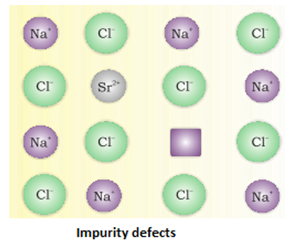• Different types of stoichiometric defects for non- ionic solids: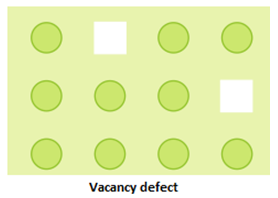• Interstitial defect: A crystal is said to have interstitial defect when some constituent particles (atoms or molecules) occupy an interstitial site. This defect results in increase in density of the substance.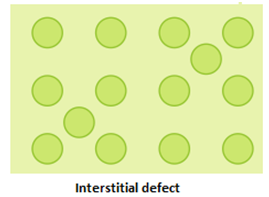• Different types of stoichiometric defects for ionic solids: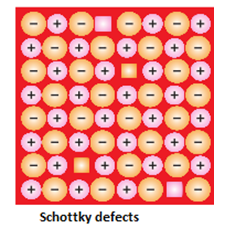• Frenkel or dislocation defect: In this defect, the smaller ion (usually cation) is dislocated from its normal site to an interstitial site. It creates a vacancy defect a tits original site and an interstitial defect a tits new location. It does not change the density of the solid. Frenkel defect is shown by ionic substance in which there is a larged difference in the size of ions. It includes ZnS,AgCl,AgBrand AgI.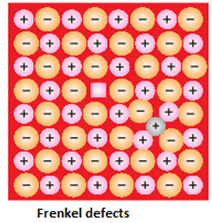• Different types of non-stoichiometric defects: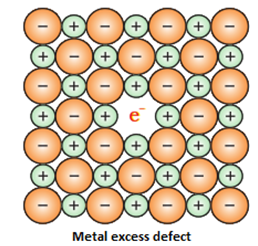• Metal deficiency: This defect arises because of absence of metal ions from its lattice sites. The electrical neutrality is maintained by an adjacention having a higher positive charge.
• Reasons for the cause of metal excess defect:

a) Anionic vacancies: A compound may have an extra metal ion if the negative ion is absent from its lattice site.This empty lattice site is called a hole.To maintain electrical neutrality this site is occupied by an electron. The hole occupied by an electron is called f-centre or Farbenz enter centre. The F- centre is responsible for the colour of the compound.

b) Presence of extracations: A compound is said to have extracations if a cation is present in the interstitial site. An electron is present in the interstitial site to maintain the electrical neutrality.

• Classification of solids based on their electrical conductivities:

a) Conductors: The solids with conductivities ranging between ${10}^{4}$ to ${10}^{7}oh{m}^{-1}{m}^{-1}$ are called conductors.

b) Insulators: These are the solids with very low conductivities ranging between ${10}^{-20}$to${10}^{-10}oh{m}^{-1}{m}^{-1}$.

c) Semi- conductors: These are the solids with conductivities in the intermediate range from${10}^{-6}$to${10}^{4}oh{m}^{-1}{m}^{-1}$.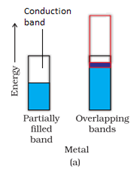b) In case of insulators, the forbidden gap is very large and the electrons are unable to excite to the conduction band.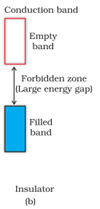c) In case of semiconductors, forbidden gap is small. Therefore, some electrons may jump to conduction band and show some conductivity. Electrical conductivity of semiconductors increases with rise in temperature, since more electron scan jump to the conduction band.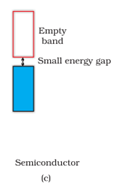• Types of semiconductors:

a) Intrinsic semiconductors: These are those semiconductors in which the forbidden gap is small. Only some electrons may jump to conduction band and show some conductivity. They have very low electrical conductivity. Example: Silicon, germanium.

b) Extrinsic semiconductors: When an appropriate impurity is added to an intrinsic semiconductor, it is called extrinsic semi conductors. Their electrical conductivity is high.

• Doping: The process of adding an appropriate amount of suitable impurity to increase the conductivity of semiconductors is known as doping.

a) The n-type semiconductors: They are formed when silicon is doped with electron rich impurity like group 15 elements. The increase in conductivity is due to the negatively charged electrons.

b) The p-type semiconductors: They are formed when silicon is doped with electron deficient impurity like group 13 elements. The increase in conductivity is due to the positively charged holes.

• Types of extrinsic semiconductors:
• Diode: It is a combination of n-type and p-type semiconductors and is used as a rectifier.
• Transistors: They are made by sandwiching a layer of one type of semiconductor between two layers of the other type of semi conductor. The npn and pnp type of transistors are used to detector amplify radio or audio signals.
• The 12- 16 compounds: These compounds are formed by the combination of group 12 and group 16 compounds.They possess an average valency of 4.Examples - ZnS,CdS,CdSe and HgTe.
• The 13- 15 compounds: These compounds are formed by the combination of group 13 and group 15 compounds.They possess an average valency of 4.Examples - InSb,AlP and GaAs.
• Every substance has some magnetic properties associated with it. The origin of these properties lies in the electrons.
• Each electron in an atom behaves like at in y magnet. Its magnetic moment originates from two types of motions:
(i) its orbital motion around the nucleus and
(ii) its spin around its own axis.
• Classification of substances based on their magnetic properties:
1. Paramagnetic substances: These are those substances which are weakly attracted by the magnetic field. It is due to presence of one or more unpaired electrons.
2. Diamagnetic substances: Diamagnetic substances are weakly repelled by a magnetic field. Diamagnetism is shown by those substances in which all the electrons are paired and there are no unpaired electrons.
3. Ferromagnetic substances: These are those substances which are attracted every strongly by a magnetic field.
4. Anti ferromagnetic substances: They have equal number of parallel and anti parallel magnetic dipoles resulting in a zero net dipolemoment.
5. Ferrimagnetic substances: They have unequal number of parallel and anti parallel magnetic dipoles resulting in an at dipole moment.